GeeksforGeeks App
Open AppBrowser
Continue

## Related Articles

• CBSE Class 11 Physics Notes

Thermodynamics is the study of Heat and Temperature and their interrelation. Thermodynamics is a branch of Physics that explains how thermal energy is changed to other forms of energy and the significance of thermal energy in matter. The behaviour of heat, work, and temperature, along with their relations to energy and entropy are governed by the Four Laws of Thermodynamics.

In this article, we will learn about the Introduction of Thermodynamics, Branches of Thermodynamics, Basic Concepts of Thermodynamics, and Examples of Thermodynamics in Daily Life.

## What is Thermodynamics?

Thermodynamics is a branch of Physcis that deals with the concept of energy, heat and temperature and their interrelation with radiation, energy and physical characteristics of matter.

The term “thermodynamics” is made of two terms, “thermo” and “dynamics” where the term “thermo” refers to heat, and the term “dynamics” refers to a mechanical motion that requires “work.”

So the field of physics that studies the relationship between heat and other types of energy is called thermodynamics.

Creating a clear boundary makes thermodynamics much simpler. The “system” refers to everything that is contained within the boundary, and the “surroundings” refers to all that is outside of it. Once the boundary diagram has been created, the flow across system boundaries can be used to describe the movement and transfer of energy. The word “universe” refers to both the surroundings and the system.

## Different  Branches of Thermodynamics

The study of Thermodynamics is classified into several branches listed below:

• Classical Thermodynamics
• Statistical Thermodynamics
• Chemical Thermodynamics
• Equilibrium Thermodynamics
• Non-Equilibrium Thermodynamics

Let’s learn about these in detail in the article.

### Classical Thermodynamics

The behaviour of matter is examined using a macroscopic perspective in classical thermodynamics. In order to determine the characteristics and predict the characteristics of the matter conducting the process, individuals take into account units like temperature and pressure.

### Statistical Thermodynamics

The development of atomic and molecular theories in the late 19th and early 20th centuries gave rise to statistical mechanics, also known as statistical thermodynamics, which added an interpretation of the microscopic interactions between individual particles or quantum-mechanical states to classical thermodynamics. This field explains classical thermodynamics as a natural consequence of statistics, classical mechanics, and quantum theory at the microscopic level. It does this by connecting the microscopic, bulk properties of materials that can be observed on the human scale to the macroscopic, individual atom, and molecule properties.

### Chemical Thermodynamics

Chemical thermodynamics is the study of how energy interacts with chemical processes or state changes in accordance with the laws of thermodynamics. Determining the spontaneity of a certain transition is the main goal of chemical thermodynamics.

### Equilibrium Thermodynamics

Equilibrium thermodynamics is the study of matter and energy transfers in systems or substances that can be moved from one state of thermodynamic equilibrium to another by agents in their environment. The phrase “thermodynamic equilibrium” refers to a condition of equilibrium in which all macroscopic flows are zero. In the case of the most basic systems or bodies, this means that their intensive properties are uniform and that their pressures are perpendicular to their boundaries. Unbalanced potentials or driving forces between the system’s macroscopically diverse components do not exist in an equilibrium state.

### Non-equilibrium Thermodynamics

Systems that are not in thermodynamic equilibrium are the focus of the field of thermodynamics known as non-equilibrium thermodynamics. The majority of systems in nature are not in thermodynamic equilibrium because they are not in stationary states and are subject to fluxes of matter and energy to and from other systems on a continual and irregular basis. More general notions than those covered by equilibrium thermodynamics are needed for the thermodynamic study of non-equilibrium systems.

## Basic Concepts of Thermodynamics

There are various concepts in thermodynamics, some of these concepts are explained as follows:

### Thermodynamic Systems

A collection of an extremely large number of atoms or molecules confined within certain boundaries such that it has certain values of pressure (P), volume (V) and temperature (T) is called a thermodynamic system.

Anything outside the thermodynamic system to which energy or matter is exchanged is called its surroundings. Taking into consideration the interaction between a system and its surroundings, a system said to be an open system if it can exchange both energy and matter with its surroundings may be divided into three classes-

• Open system: A system is said to be an open system if it can exchange energy and matter with its surroundings.
• Closed system: A system is said to be a closed system if it can exchange only energy (not matter) with its surroundings.
• Isolated system:  A system is said to be isolated if it can neither exchange energy nor matter with its surroundings.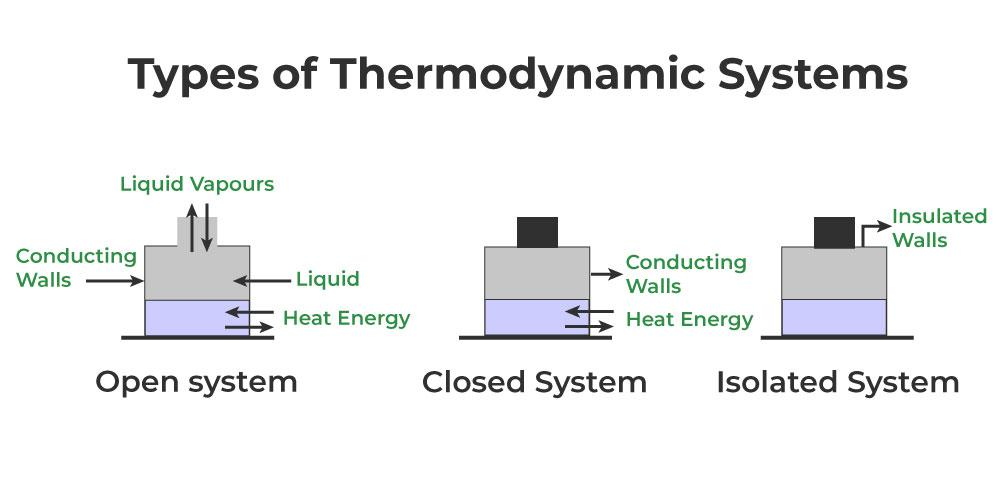Type of Systems

### Surroundings

The term “surroundings” refers to everything outside the system that affects how it behaves. There is a boundary separating the system from its surroundings. It could be stationary, mobile, or fictitious. It won’t take up any space in terms of mass or volume. For example, consider a closed beaker with liquid inside as shown below. The liquid inside the beaker is the system, while the outline of the beaker represents the boundary of the system. And matter outside the system and boundary is called its surroundings.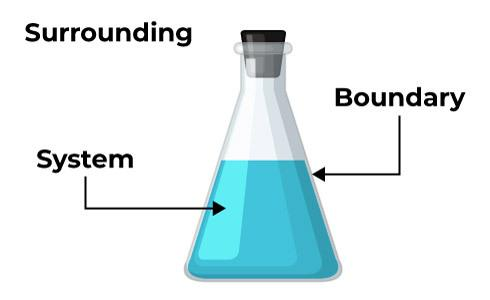System and Surroundings

### Heat

Heat is energy that is transmitted between objects or systems as a result of a temperature difference. Heat is conserved energy, which means it cannot be created or destroyed. However, it can be moved from one location to another. Additionally, heat can be transformed into and out of various types of energy.

### Work

The work done by a system or on a system during a process depends not only on the system’s starting and final states but also on the path chosen for the process. When a force acting on a system moves the body in its own direction, work has been done. Force and displacement combine to create the work (W) that is done to or by a system.

### Internal Energy

The kinetic and potential energies of the molecules are added up to form internal energy. The system’s internal energy is represented by the letter U. Kinetic energy is the energy that molecules or atoms possess due to their motion. Two molecules have some potential energy because they are attracted to one another. The total kinetic and potential energy of the atoms or molecules that make up a system is what is known as the system’s internal energy.

## Thermodynamic Properties or Variables

A thermodynamic system can be described by specifying its pressure, volume, temperature, internal energy, enthalpy, and the number of moles. These parameters or variables are called thermodynamic variables.

Thus, the variables which are required to specify the state of the thermodynamic system are called thermodynamic variables. Entropy is a measurement of energy present in a system or process but is not available to do work. It is also defined as the measure of disorder in the system. Enthalpy is the measurement of the total energy of a thermodynamic system.

Types of Thermodynamic Variables

• Intensive variables: The variables which are independent of the size of the system are called intensive variables. e.g. Temperature, pressure, and specific beat capacity.
• Extensive variables: The variables which depend on the size or mass of the system are called extensive variables. e.g. Volume, energy, entropy, heat capacity, and enthalpy.

## Thermodynamic Equilibrium

Thermodynamic equilibrium is a state of a system in which there is no net change occurs within the system over time i.e., the state of a thermodynamic system in which macroscopic properties such as temperature, pressure, and chemical composition, remain constant. There are different types of thermodynamic equilibrium:

• Thermal Equilibrium: In the state of thermal equilibrium, the temperature of the system remains constant i.e., there is no net heat transfer occurring within the system.
• Mechanical Equilibrium: When in a system either there is no net force acting or there is no pressure difference, then this state of the system is called mechanical equilibrium. This state is also sometimes referred to as a state of mechanical balance.
• Chemical Equilibrium: A thermodynamic system is said to be in chemical equilibrium when the rates of forward and reverse reactions become equal. When the system reaches chemical equilibrium, the concentrations of reactants and products remain constant over time.
• Phase Equilibrium: A system is said to be in Phase equilibrium when different phases of a substance such as solid, liquid, and gas, coexist in a system at the same time. In other words, the system is in phase equilibrium when the rates of phase changes, such as evaporation and condensation, are equal.

## Thermal Equilibrium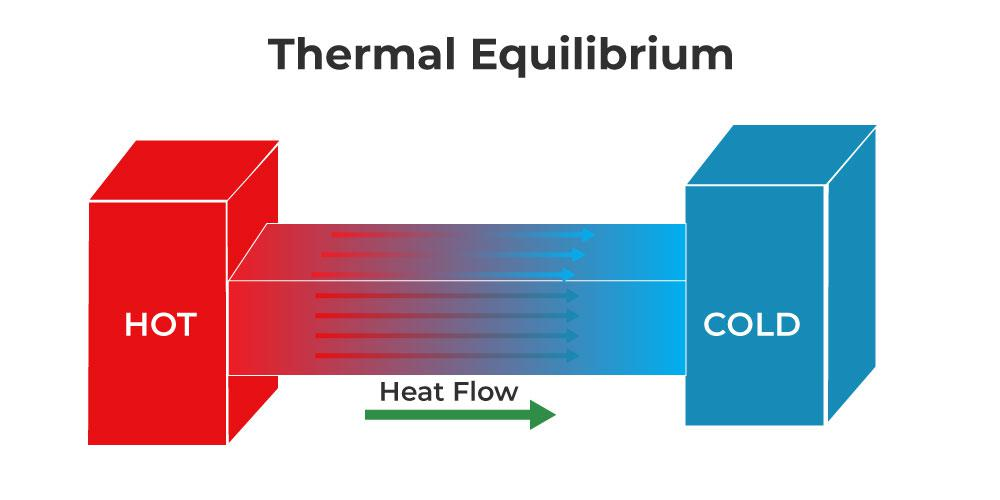thermal Equilibrium

When two bodies having different temperatures are placed in contact, then the energy flows from a body at a higher temperature to a body at a lower temperature. The flow of energy continues from one body to another to attain the same temperature. When both the bodies in contact have the same temperature and there is no energy for the body till both bodies are between them, then these bodies are in thermal equilibrium. Thus, two bodies or systems in contact are said to be in thermal equilibrium if both are at the same temperature.

• A thermodynamic system is said to be in thermodynamic equilibrium if its variables like pressure, volume, temperature, number of particles, etc. do not change with time.
• Any isolated system is in thermodynamic equilibrium.

### Thermodynamic Processes

Any process in which the thermodynamic variables of a thermodynamic system change is known as the thermodynamic process.

• Quasi-Static Process (Quasi-Static means nearly static): A process in which the system departs only infinitesimally from the equilibrium state is known as a quasi-static process In this process, the change in pressure or change in volume or change in temperature of the system is very very small.
• Isothermal Process: A process in which the pressure and volume of the system change at constant temperature are called the isothermal process. In this case, P and V change but T is constant. i.e. dT (change in temperature) = 0.
• Adiabatic Process: A process in which pressure, volume, and temperature of the system change, but there is no exchange of heat between the and its surroundings is called the adiabatic process. In this case, P. V and T change but Q = 0. The system should be compressed or allowed to expand suddenly so that there is no time for the exchange of heat between the system and its surroundings. Since these two conditions are not fully realized in practice, so no process is perfectly adiabatic.
• Isochoric Process:  A thermodynamic process that takes place at constant volume is called the isochoric process. It is also known as the isovolumic process. In this case, dV = 0.
• Isobaric Process: A thermodynamic process that takes place at constant pressure is called the isobaric process. In this case, dP = 0.
• Cyclic Process: A cyclic process consists of a series of changes that return the system to its initial state.

## Thermodynamic Potentials

The stored energy in a system is measured by its thermodynamic potentials. Potentials measure how a system’s energy transforms from its initial state to its final one. Depending on the constraints of the system, such as temperature and pressure, different potentials are used.

Different forms of thermodynamic potentials are mentioned below:

• Internal Energy (U): It is equal to the sum of the ability to do work and the ability to release heat.
• Gibbs Energy (G): It is the ability to do non-mechanical work.
• Enthalpy (H): It is the ability to do non-mechanical work and the ability to emit heat.
• Helmholtz Energy (F): It is the ability to do both mechanical and non-mechanical work.

### What is Enthalpy?

In a thermodynamic system, energy is measured by enthalpy. Enthalpy is a measure of a system’s total heat content and is equal to the system’s internal energy plus the sum of its volume and pressure. Enthalpy is a property or state function that resembles energy; it has the same dimensions as energy and is therefore measured in joules or ergs. The value of enthalpy is entirely dependent on the temperature, pressure, and composition of the system, not on its history.

### What is Entropy?

Entropy is the measurement of the amount of thermal energy per unit of temperature in a system that cannot be used for useful work. Entropy is a measure of a system’s molecular disorder or randomness since work is produced by ordered molecular motion. Entropy theory offers a deep understanding of the direction of spontaneous change for many common events.

## Laws of Thermodynamics

Thermodynamic systems at thermal equilibrium are characterized by fundamental physical constants like energy, temperature, and entropy, which are defined by thermodynamic laws. These thermodynamic principles describe how these quantities act in different situations.

### Zeroth Law of Thermodynamics

According to the Zeroth Law of Thermodynamics, if two bodies are separately in thermal equilibrium with a third body, then the first two bodies are likewise in thermal equilibrium with each other. This indicates that if system A is in thermal equilibrium with system B, and system C is likewise in thermal equilibrium with system B, then both systems A and C are in thermal equilibrium.

### First Law of Thermodynamics

Energy cannot be generated or destroyed, according to the first law of thermodynamics, but it can be converted from one form to another. Heat, internal energy, and work are all addressed by the first law of thermodynamics. Energy cannot be generated or destroyed, according to the first law of thermodynamics, but it can be converted from one form to another. According to this law, some of the heat provided to the system is utilized to change the internal energy, while the remaining is used to perform work.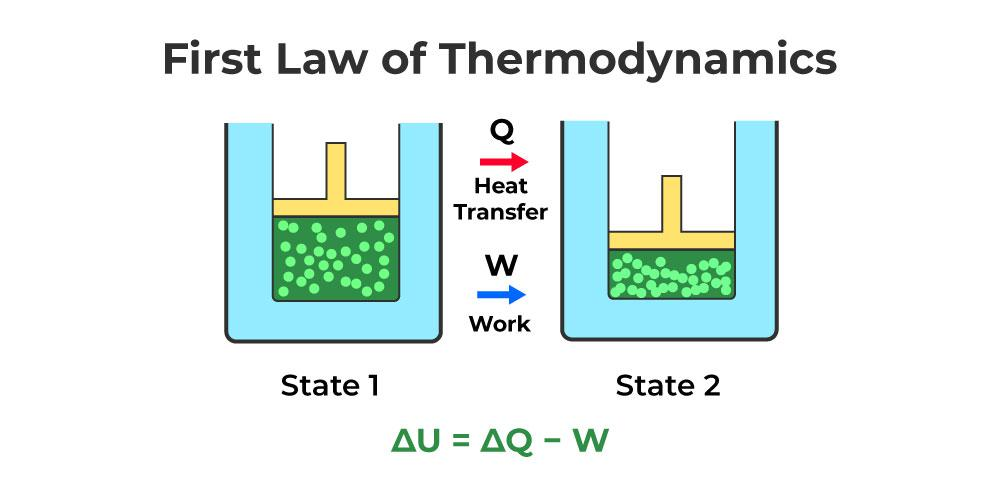Mathematically, it may be expressed as

ΔQ = ΔU + W

Where,

• The heat given or lost is denoted by ΔQ.
• The change in internal energy is denoted by ΔU.
• W stands for work done.

The above equation can alternatively be written as follows:

ΔU = ΔQ − W

As a result of the above equation, we may deduce that the quantity (ΔQ – W) is unaffected by the path taken to change the state. Furthermore, when heat is applied to a system, internal energy tends to rise, and vice versa.

### Second Law of Thermodynamics

In an isolated system, the second law of thermodynamics asserts that entropy always increases. Any isolated system progresses spontaneously toward thermal equilibrium or the state of maximum entropy. The universe’s entropy is always increasing and never decreasing.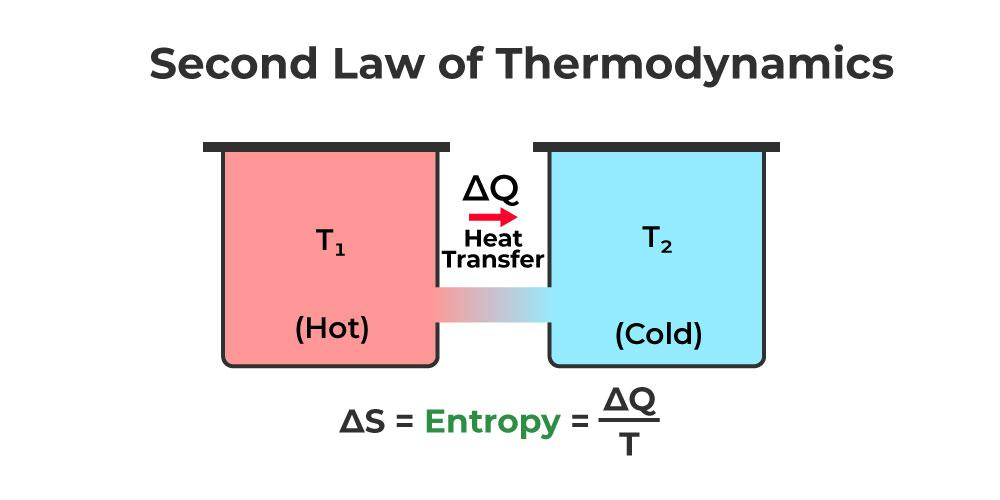### Third Law of Thermodynamics

The third law of thermodynamics states that when the temperature approaches absolute zero, the entropy of a system approaches a constant value. At absolute zero temperature, the entropy of a pure crystalline solid is zero. If the perfect crystal has only one state with minimum energy, this assertion holds true.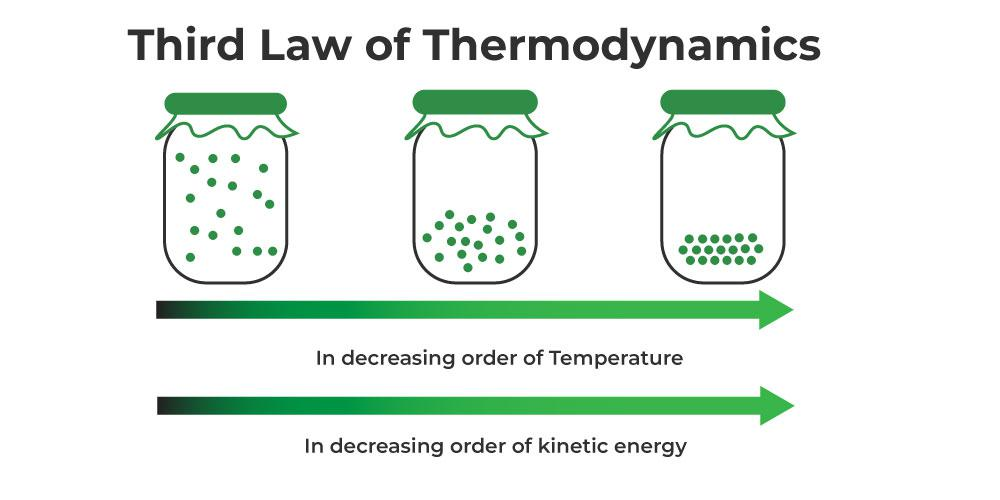## Thermodynamics Examples in Daily Life

We came across various examples in our daily life which can be explained using thermodynamic properties. Some of them are,

• Melting of Ice Cubes: Drinks with ice cubes become cooler as the heat from the drink is absorbed. If we neglect to drink it, it will eventually warm back up to room temperature by absorbing heat from the environment. The first and second laws of thermodynamics govern how all of this works.
• Sweating in a Crowded Room: In a crowded room, everyone begins to sweat. By transmitting body heat to the sweating, the body begins to cool down. Sweat evaporates, heating the space. Again, this occurs as a result of the application of the first and second laws of thermodynamics. Keep in mind that heat is not lost but rather moved until equilibrium is reached with the least amount of entropy.
• Flipping a Light Switch: Different kinds of power plants, including thermal and nuclear ones, are studied using thermodynamics.

## Solved Problems on Thermodynamics

Example 1: Calculate ΔG at 280 K for the reaction,

2NO + O2 –> 2NO2

when ΔH and ΔS of the reaction are -100 J and -0.25 J/K respectively

Solution:

Given,

ΔH = -100 J
ΔS = -0.25 J/K
T = 280 K

We know that,

ΔG = ΔH – TΔS
⇒ ΔG = (-100) -280(0.25)
⇒  ΔG = -100 – 70
⇒  ΔG = -170 J

Example 2: Calculate the temperature at which ΔG of the given reaction is 200 J when ΔH and ΔS of the reaction are -150 J and -0.5 J/K respectively

H2 + I2 –> 2HI

Solution:

Given,

ΔG = 200 J
ΔH = -150 J
ΔS = -0.5 J/K

T =?

We know that,

ΔG = ΔH – TΔS

Thus,

⇒ 200 = (-150) – T(-0.5)

⇒ 200 = -150 + T/2

⇒ 200 + 150 = T/2

⇒ T = 700 K

Example 3: If an ideal heat engine operates in a Carnot cycle between 600 K and 400 K and if it absorbs 6000 J of heat at a higher temperature then find the heat supplied from the source.

Solution:

Given,

T1 = 600 K

T2 = 400 K

Heat Absorbed at High Temperature = 6000 J

Heat Supplied from Source =?

Efficiency of Heat Engine (E) = 1 – (T2 / T1)

⇒ Efficiency of Heat Engine (E) = 1 – 400/600 = 1- 2/3
⇒ Efficiency of Heat Engine (E) = 1/3

We know that,

E = Heat Supplied from Source/Heat Absorbed at High Temperature

⇒1/3 = Heat Supplied from Source/6000

Heat Supplied from Source = 2000 J

Example 4: Find the Efficiency of the Heat Engine if it operates between 700 K and 350 K.

Solution:

T1 = 700 K

T2 = 350 K

Efficiency of Heat Engine (E) = 1 – (T2 / T1)

⇒ Efficiency of Heat Engine (E) = 1 – 350/700 = 1- 1/2

⇒ Efficiency of Heat Engine (E) = 1/2

⇒ E = 1/2 × 100 % = 50%

## FAQs on Thermodynamics

### Q1: What is an Isolated system?

A system is said to be isolated if it can neither exchange energy nor matter with its surroundings.

### Q2: What is the significance of Thermodynamics Laws?

Temperature, energy, and entropy, which describe the physical quantities that define thermodynamic systems at thermal equilibrium, are defined by the laws of thermodynamics.

### Q3: Can energy be lost or destroyed?

No, the energy can not be destroyed and created. But it can only be transferred from one form of energy to other.